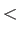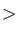### Keyword MATINV

The matrix inversion technique for the Coulomb matrix in the variational density fitting is selected with this keyword.
Options:
NUMERICAL / ANALYTICAL
 NUMERICAL Iterative calculation of the fitting coefficients is specified. This is the default. ANALYTICAL Analytical calculation of the fitting coefficients is specified.
SVD / LUD / ONE
 SVD Use singular value decomposition for the calculation of the inverse of the Coulomb matrix. This is the default. LUD Use Cholesky decomposition for the calculation of the inverse of the Coulomb matrix. ONE Use the identity matrix as initial approximation to the inverse of the Coulomb matrix. Only meaningful in combination with the NUMERICAL option. DIAGONAL Performs the calculation of the fitting coefficients in the diagonal basis. TOL=RealTolerance for the singular value decomposition. The default is. MAX=IntegerMaximum number of iteration cycles for the iterative calculation of the fitting coefficients. The default is 5000.
Description:
Calculation of the Coulomb and, in the case of ADFT, exchange-correlation fitting coefficients requires the solution of linear equation systems with the dimension of the auxiliary function set. For very large systems this task can represent a computational bottleneck. With the options NUMERICAL and ANALYTICAL of the MATINV keyword, numerical or analytical algorithms [25,192] for the solution of the fitting equation systems are requested. Whereas analytic solutions must be initialized at each geometry, numerical solutions need only be initialized at the first geometry . The actual algorithm is selected with the options SVD, LUD, or ONE. The SVD and LUD options work with both numerical and analytical algorithms. The option SVD specifies singular value decomposition of the Coulomb matrix. This involves the diagonalization of that matrix, either for the first geometry only, if used in combination with NUMERICAL, or for each geometry if used in combination with ANALYTICAL. SVD is particularly recommended if large auxiliary function sets such as GEN-A2*, GEN-A3*, or GEN-A4* are used. With the option LUD, the inverse Coulomb matrix is calculated via Cholesky decomposition . This approach avoids matrix diagonalization and is, therefore, well-suited for large systems with medium-sized auxiliary function sets such as A2 or GEN-A2. The option ONE is meaningful only for the numerical approach. Here the inverse Coulomb matrix is initialized as a unit matrix and then updated during the first SCF. This is the least memory-demanding approach available in deMon2k.

The options DIAGONAL and TOL of the keyword MATINV are meaningful only in combination with the SVD option. In the DIAGONAL SVD approach, eigenvalues and eigenvectors are quenched  and the equation systems are always solved in this diagonal representation. This is the numerically most stable approach. Thus, we recommend the use of,

``` MATINV SVD DIAGONAL ANALYTICAL
```

if significantly large negative MinMax errors hamper SCF convergence. The SVD threshold can be modified with the TOL option. The default value ofhas proven to be robust for most applications. Reducing this value usually will complicate SCF convergence. Note that calculations with different SVD thresholds are not compatible with each other! The option MAX of the MATINV keyword specifies the maximum number of iterative fitting cycles and is, therefore, only meaningful in combination with the option NUMERICAL. The convergence of the numerical fitting can be printed by using PRINT FIT (see 4.12.2) in the input.